PROBLEM #1, SET 2 – NUMBERS

1. How deep would the water in a trench three inches wide and
sixteen feet long have to be to contain 24.0 cubic feet of
water?

This problem is likely best done with W5P method because it asks for a
length when you are given two other lengths and a volume. All units are in
English system.

GIVEN:   l1
= 16 ft.,       l2 = 3 in,
l = 24.0 ft3
You know this from the
units and dimensions table in the Units section of Lessons.

FIND:   a length, l
You know the symbol for length from the
units and dimensions table in the Units section of Lessons.

FORMULA:   V = l l l
You know two lengths and a volume. You are asked to find a
length. The volume formula must be the one you need because it is the relationship
among three lengths and a volume. You know this from the list of formulas in the
formula section of Units in Lessons.

SOLVE: Using
algebra, solve for the length you are looking for. Once you have one of the
lengths on one side alone, substitute the known values from
GIVEN into the working equation,
simplify, and do the math. Here the math includes some DA to get the units right.

WHAT YOU WRITE DOWN SHOULD LOOK LIKE THIS:

GIVEN:   l1
= 16 ft.,       l2 = 3 in,
V = 24.0 ft3
FIND:   a length, l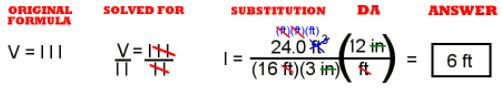The calculator math is:   24 ÷ 16 ÷ 3 x 12 =

show the raw answer (the units that remain and the number on your calculator).
Change the raw answer to the correct number of significant digits and change into
scientific notation if the answer is greater than 1000 or less than 1/1000. In this case,
no change is needed.

PROBLEM #2, SET 2 – NUMBERS

2. Cloth is four feet wide as it comes on the bolt. What
length of cloth would you need to cover a surface of 58.0 square
yards?

This problem is likely best done with W5P method because it asks for a
length when you are given one other length and an area. All units are in
English system.

GIVEN:   l1
= 4 ft.,     A = 58.0 ft2
You know this from the
units and dimensions table in the Units section of Lessons.

FIND:   a length, l
You know the symbol for length from the
units and dimensions table in the Units section of Lessons.

FORMULA:   A = l l
You know one length and an area. You are asked to find a
length. The area formula must be the one you need because it is the relationship
among two lengths and a volume. You know this from the list of formulas in the
formula section of Units in Lessons.
State the original formula here.

SOLVE: Using
algebra, solve for the length you are looking for. Once you have one of the
lengths on one side alone, substitute the known values from
GIVEN into the working equation,
simplify, and do the math. Here the math includes some DA to get the units right.

WHAT YOU WRITE DOWN SHOULD LOOK LIKE THIS:
GIVEN:   l1
= 4 ft.,     A = 58.0 ft2
FIND:   a length, l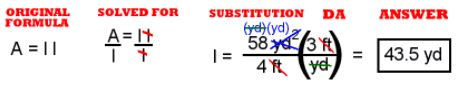The calculator math is:   58 ÷ 4 x 3 =

show the raw answer (the units that remain and the number on your calculator).
Change the raw answer to the correct number of significant digits and change into
scientific notation if the answer is greater than 1000 or less than 1/1000. No
change to the raw answer is needed in this problem.

PROBLEM #3, SET 2 – NUMBERS

3. It is 762 miles from here to Chicago. An obese Chemistry
teacher jogs at a rate of one mile every twenty minutes.
How long would it take him to jog to Chicago if he jogged
continuously and REALLY wanted to get there?

This problem is likely best done with W5P method because it asks for a
time when you are given a length and a velocity. All units are in
English system.

GIVEN:   d* = 762 miles,     v = 1 mile/20 min
You know this from the
units and dimensions table in the Units section of Lessons.
*
I will admit to some sneakiness here. The dimension of distance has more than
one symbol. Seeing the velocity and distance in the problem, I chose the symbol
for distance that goes with the formula.

FIND:   a time, t
The question asks, "How long would it take …," which asks for a time.
You know the symbol for time from the
units and dimensions table in the Units section of Lessons.

FORMULA:   v t = d
You know a length and a velocity. You are asked to find a
time. The rate equation must be the one you need because it is the relationship
among a rate (or velocity), a time, and a distance. You know this from the list of formulas in the
formula section of Units in Lessons.

SOLVE: Using
algebra, solve for the length you are looking for. Once you have the time on
one side alone, substitute the known values from
GIVEN into the working equation,
simplify, and do the math. Here the math includes some DA to get the time units manageable.

WHAT YOU WRITE DOWN SHOULD LOOK LIKE THIS:

GIVEN:   d = 762 miles,     v = 1 mile/20 min

FIND:   a time, t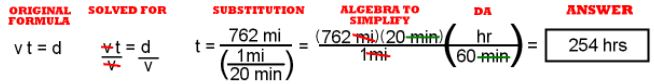The calculator math is:   762 x 20 ÷ 60 =

show the raw answer (the units that remain and the number on your calculator).
Change the raw answer to the correct number of significant digits and change into
scientific notation if the answer is greater than 1000 or less than 1/1000.
Here, because of the W5P, there is no need to change the raw answer.

PROBLEM #4, SET 2 – NUMBERS

4. The density of lead is 11.4 g/cc. What is the mass of a
block of lead two cm by ten cm by four cm?

This problem is likely best done with W5P method because it asks for a
mass when you are given three lengths and a density. All units are in
metric system.

GIVEN:
l1 = 2 cm,
l2 = 10 cm,
l3 = 4 cm,     D = 11.4 g/cc
You know this from the
units and dimensions table in the Units section of Lessons.

FIND:   a mass, m
The question asks, "What is the mass …," which asks for m, the mass.
You know the symbol for mass from the
units and dimensions table in the Units section of Lessons.

FORMULAS:   V = l l l     D = m/V
You know three lengths and a density. You are asked to find a
mass. For this question, you need two of the equations from the
formula section of Units in Lessons, the
formula for volume and the density formula.

SOLVE: Using
algebra, solve for the length you are looking for. Once you have the time on
one side alone, substitute the known values from
GIVEN into the working equation,
simplify, and do the math. Here the math includes some DA to get the units right.

WHAT YOU WRITE DOWN SHOULD LOOK LIKE THIS:

GIVEN:
l1 = 2 cm,
l2 = 10 cm,
l3 = 4 cm,     D = 11.4 g/cc

FIND:   a mass, m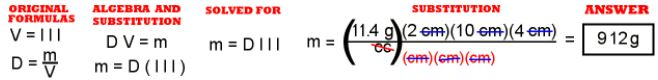The calculator math is:   11.4 x 2 x 10 x 4 =

show the raw answer (the units that remain and the number on your calculator).
Change the raw answer to the correct number of significant digits and change into
scientific notation if the answer is greater than 1000 or less than 1/1000. Once
again there is no need to change the raw answer.

PROBLEM #5, SET 2 – NUMBERS

5. How many liters of water could you fit into a container
measuring 10.0 ft by 13.0 ft by 8.00 ft?

This problem is likely best done with W5P method because it asks for a
volume when you are given three lengths. The answer is asked for in metric system,
but the information has been given in English system, so the units will need some DA.

GIVEN:
l1 = 10.0 ft,
l2 = 13.0 ft,
l3 = 8.00 ft,
You know this from the
units and dimensions table in the Units section of Lessons.

FIND:   a volume, V
The question asks for a volume, V.
You know the symbol for volume from the
units and dimensions table in the Units section of Lessons.

FORMULA:   V = l l l
You know three lengths. You are asked to find a volume.
For this question you need the volume equation from the
formula section of Units in Lessons.

SOLVE:
The equation is already solved for V, the volume. Substitute the known values from
GIVEN into the working equation,
simplify, and do the math. Here the math includes some DA to get the units right.

WHAT YOU WRITE DOWN SHOULD LOOK LIKE THIS:

GIVEN:
1 = 10.0 ft,
2 = 13.0 ft,
3 = 8.00 ft,

FIND:   a volume, V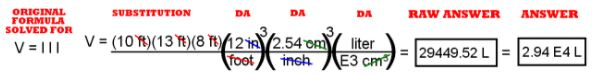By calculator it is:   10 x 13 x 8 x 12 x 12 x 12 x 2.54 x 2.54 x 2.54 ÷ 1000 =
Likely, you know some ways to make the math shorter.

show the raw answer (the units that remain and the number on your calculator).
Change the raw answer to the correct number of significant digits and change into
scientific notation if the answer is greater than 1000 or less than 1/1000.

PROBLEM #6, SET 2 – NUMBERS

6. A box of qrtpsdfgh measures 24.0 x 4.00 x 12.0 inches. It
has a density of 38.0 pounds per cubic foot. What is the
weight of qrtpsdfgh in the box? (Notice the English system
density!)

This problem is likely best done with W5P method because it asks for a
weight when you are given three lengths and a weight density. The units are all
English system.

GIVEN:
l1 = 24.0 in,
l2 = 4.00 in,
l3 = 12.0 in,
D = 38.0 #/cu.ft.
You know this from the
units and dimensions table in the Units section of Lessons.

FIND:   a weight, Fw
The question asks for a weight, Fw.
You know the symbol for weight from the
units and dimensions table in the Units section of Lessons.

FORMULAS:
D = Fw/V     V = l l l
You know three lengths and a weight density. You are asked to find a weight.
The formulas come from the
formula section of Units in Lessons.

SOLVE:
The equation is already solved for V, the volume. Substitute the known values
from GIVEN into the working equation, simplify,
and do the math. Here the math includes some
DA to get the units right.

WHAT YOU WRITE DOWN SHOULD LOOK LIKE THIS:

GIVEN:
1 = 24.0 in,
2 = 4.00 in,
3 = 12.0 in,
D = 38.0 #/cu.ft.

FIND:   a weight, Fw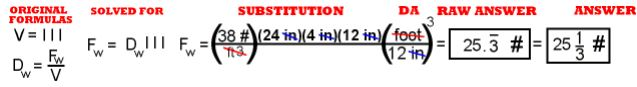The calculator math is:   38 x 24 x 4 x 12 ÷ 12 ÷ 12÷ 12 =   or just:
38 x 24 x 4 ÷ 12÷ 12 =

show the raw answer (the units that remain and the number on your calculator).
Change the raw answer to the correct number of significant digits and change into
scientific notation if the answer is greater than 1000 or less than 1/1000.

PROBLEM #7, SET 2 – NUMBERS

7. My basement was flooded after a big rain. The basement is
rectangular 12.0 ft by 17.0 ft. The water was measured
as it drained out at 51.0 cubic feet. How deep was the water in
the basement?

This problem is likely best done with W5P method because it asks for a
distance when you are given two lengths and a volume. The units are all
English system.

GIVEN:
1 = 12.0 ft,
2 = 17.0 ft,
V = 51.0 cu.ft.
You know this from the
units and dimensions table in the Units section of Lessons.

FIND:   a length, l
The question asks for a length, l.
You know the symbol for length from the
units and dimensions table in the Units section of Lessons.

FORMULA:   V = l l l
You know two lengths and a volume. You are asked to find a length.
The formulas come from the
formula section of Units in Lessons.

SOLVE:
Solve for one of the lengths. Substitute the known values from
GIVEN into the working equation,
simplify, and do the math. Here the math includes some
DA to get the units right.

WHAT YOU WRITE DOWN SHOULD LOOK LIKE THIS:

GIVEN:
1 = 12.0 ft,
2 = 17.0 ft,
V = 51.0 cu.ft.

FIND:   a length, l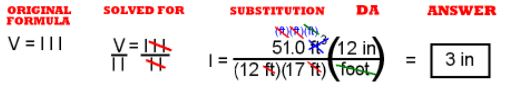The calculator math is:   51 ÷ 12 ÷ 17 x 12 =   or just:   51 ÷ 17 =

show the raw answer (the units that remain and the number on your calculator).
Change the raw answer to the correct number of significant digits and change into
scientific notation if the answer is greater than 1000 or less than 1/1000. No change
from the raw answer is needed here.

PROBLEM #8, SET 2 – NUMBERS

8. A standard expressway lane is 11.0 ft wide. How long would
a four lane expressway be if it had a square mile of
pavement?

This problem is likely best done with W5P method because it asks for a
distance when you are given one length and an area. The units are all
English system.

GIVEN:
1 = 44.0 ft(4 lanes at 11 ft each!),
= 1 sq. mi.
You know this from the
units and dimensions table in the Units section of Lessons.

FIND:   a length, l
The question asks for a length, l.
You know the symbol for length from the
units and dimensions table in the Units section of Lessons.

FORMULA:  A = l l
You know one length and an area. You are asked to find a length. The formulas come from the formula section of Units in Lessons.

SOLVE:
Solve for one of the lengths. Substitute the known values from
GIVEN into the working equation,
simplify, and do the math. Here the math includes some DA to get the units right.

WHAT YOU WRITE DOWN SHOULD LOOK LIKE THIS:

GIVEN:
1 = 44.0 ft,     A
= 1 sq. mi.

FIND:   a length, l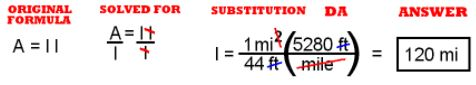The calculator math is:   5280 ÷ 44 =

show the raw answer (the units that remain and the number on your calculator).
Change the raw answer to the correct number of significant digits and change into
scientific notation if the answer is greater than 1000 or less than 1/1000.
The raw answer needs no change to it.

PROBLEM #9, SET 2 – NUMBERS

9. The density of gasoline is 720 g per liter. What is the
mass of a jar of gasoline three cm by four cm by five cm?

This problem is likely best done with W5P method because it asks for a
density when you are given three lengths and a mass. The units are all
metric.

GIVEN:
l1 = 3 cm,
l2 = 4 cm,
l3 = 5 cm,
D = 720 g/L
You know this from the
units and dimensions table in the Units section of Lessons.

FIND:   mass, m
The question asks for a mass, m.
You know the symbol for length from the
units and dimensions table in the Units section of Lessons.

FORMULA(S):  V = l l l     D = m/V
You know three lengths and a density. You are asked to find a mass.
The formulas come from the
formula section of Units in Lessons.

SOLVE:
Solve for the mass. Substitute the known values from
GIVEN into the working equation,
simplify, and do the math. Here the math includes some DA to get the units right.

WHAT YOU WRITE DOWN SHOULD LOOK LIKE THIS:

GIVEN: 1 = 3 cm,
2 = 4 cm,
3 = 5 cm,
D = 720 g/L

FIND:   a length, l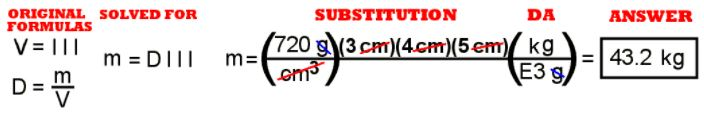The calculator math is:   720 x 3 x 4 x 5 ÷ 1000 =

show the raw answer (the units that remain and the number on your calculator).
Change the raw answer to the correct number of significant digits and change into
scientific notation if the answer is greater than 1000 or less than 1/1000.
Once again, the raw answer does not need to be changed.

PROBLEM #10, SET 2 – NUMBERS

10. How many liters of water could you fit into a hot tub that
measures 1.50m deep and three meters in diameter? How
many people?
(1 cubic meter = 1000L)

This problem is likely best done with W5P method because it asks for the
volume of a cylinder when you are given a length as height and another length as diameter. The units are all
metric.

GIVEN:
= 11.0 ft,
d = 3 m (so the radius, r = 1.5 m)
You know this from the
units and dimensions table in the Units section of Lessons.

FIND:   a volume, V
The question asks for a length, l.
You know the symbol for volume from the
units and dimensions table in the Units section of Lessons.

FORMULA:
Vc = pi r2
You know the radius and height of a cylinder. You are asked to find the volume.
The formula comes from the
formula section of Units in Lessons.

SOLVE:
The volume is already solved for in the memorized equation.
GIVEN into equation and do the math. Here the math includes some DA to get the units right.

WHAT YOU WRITE DOWN SHOULD LOOK LIKE THIS:

GIVEN:
= 11.0 ft,
d = 3 m (so the radius, r = 1.5 m)

FIND:   a volume, V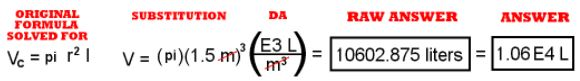The calculator math is:   pi x 1.5 x 1.5 x 1.5 x 1000 =

show the raw answer (the units that remain and the number on your calculator).
Change the raw answer to the correct number of significant digits and change into
scientific notation if the answer is greater than 1000 or less than 1/1000.
You can leave the raw answer as it is.

The answer to the second question is:   THE MORE, THE MERRIER.

PROBLEM #11, SET 2 – NUMBERS

11. Mr. Richland has a swimming pool 10.0 ft by 20.0 ft. He
had a wild party and they threw his son’s Volkswagen into
the pool. The VW has a volume of 10 cubic feet. It was sealed up,
but it sank because of all the lead bricks in it. (?!?!?*?*?)
(a) What is the area of the pool? (b) How much did the VW
raise the water level?

A major idea here is that the volume of the VW is equal to the volume of
displaced water. The displaced water can be measured as a layer on top of the
other water in the pool.

Part (a) of the problem is easy and intuitive. Given two distances, what is the area?
Given = 10 ft and = 20 ft,
= = (10 ft)(20 ft) = 200 ft2

The second part of this problem is likely best done with W5P method because it asks for a
distance when you are given two lengths and a volume. The units are all English system.

GIVEN:
1 = 10.0 ft,
2 = 20.0 ft,
V
= 10 cu. ft.
You know this from the
units and dimensions table in the Units section of Lessons.

FIND:   a length, l
The question asks for a length, l.
You know the symbol for length from the
units and dimensions table in the Units section of Lessons.

FORMULA:  V = l l l
You know two lengths and a volume. You are asked to find a length.
The formulas come from the
formula section of Units in Lessons.

SOLVE:
Solve for one of the lengths. Substitute the known values from
GIVEN into the working equation,
simplify, and do the math. Here the math includes some DA to get the units right.

WHAT YOU WRITE DOWN SHOULD LOOK LIKE THIS:

GIVEN:
1 = 10.0 ft,
2 = 20.0 ft,
V
= 10 cu. ft.

FIND:   a length, l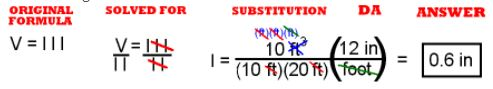The calculator math is:   10 ÷ 10 ÷ 20 x 12 =   or just:   12 ÷ 20 =